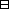## Group Theory and Linear Algebra

Last updated: 21 September 2014

## Lecture 10: Eigenvectors and annihilators

Let $V$ be a vector space over $ℂ\text{.}$ Let $f:V\to V$ be a linear transformation.

The $ℂ\left[t\right]\text{-module}$ defined by $f$ is the vector space $V$ with $ℂ\left[t\right]\text{-action}$ given by $p·v=(a0+a1f+⋯+aNfN)v$ if $v\in V$ and $p={a}_{0}+{a}_{1}t+{a}_{2}{t}^{2}+\cdots +{a}_{N}{t}^{N}\in ℂ\left[t\right]\text{.}$

$V= { (a1a2a3a4a5) | ai∈ℂ }$ has basis $B= { (10000), (01000), (00100), (00010), (00001), }$ and the matrix $Bf= ( 21000 02100 00200 00051 00005 )$ defines $f:V\to V\text{.}$ Then $(3t+6t3)· (a1a2a3a4a5) = ( 3 ( 21 21 2 51 5 ) +6 ( 21 21 2 51 5 ) 3 ) (a1a2a3a4a5) = …$

An $f\text{-invariant}$ subspace, or $ℂ\left[t\right]\text{submodule,}$ of $V$ is a subspace $W\subseteq V$ such that if $w\in W$ then $fw\in W\text{.}$

Let $\lambda \in ℂ\text{.}$ The $\lambda \text{-eigenspace}$ of $f$ is $Vλ={v∈V | fv=λv}.$ An eigenvector with eigenvalue $\lambda$ is a vector $v\in {V}_{\lambda }\text{.}$

In our previous example, $( 21 21 2 51 5 ) (70000)= (140000)= 2·(70000).$ So $(70000)∈V2.$ $( 21 21 2 51 5 ) (00010)= (00050)= 5· (00010).$ So $(00010)∈V5.$ $( 21 21 2 51 5 ) (00100)= (01200)≠ 2· (00100).$ So $(00100) ∉V2.$

The $\lambda \text{-generalized}$ eigenspace of $V$ is $Vλgen= {v∈V | there exists k∈ℤ>0 with (f-λ)kv=0}.$ In our previous example, $f-2 = ( 21 21 2 51 5 ) -2 ( 1 1 1 1 1 ) = ( 01 01 0 31 3 ) , (f-2)2 = ( 001 00 0 96 9 ) , (f-2)3 = ( 000 00 0 81108 81 )$ and $(f-2)3 (a1a2a300) = (00000)$ if ${a}_{1},{a}_{2},{a}_{3}\in ℂ\text{.}$ So $V2gen⊇ { (a1a2a300) | a1,a2,a3∈ℂ } .$

The annihilator of $V$ is $ann V= { p∈ℂ[t] | if v∈V then pv=0 } .$ The minimal polynomial of $f$ is $m\in ℂ\left[t\right]$ such that $ann V=mℂ[t]$ where $mℂ\left[t\right]=\left\{mq | q\in ℂ\left[t\right]\right\}=\left\{\text{multiples of} m\right\}\text{.}$

In our previous example, $(f-2)3 = ( 000 00 0 81108 81 ) , (f-5)2 = ( 9-61 9-6 9 00 00 )$ and $(f-2)3 (f-5)2= ( 000 000 000 00 00 ) .$ So ${\left(t-2\right)}^{3}{\left(t-5\right)}^{2}\in \text{ann} V\text{.}$

Let $f:V\to V$ be a linear transformation.

 (a) Let $\lambda \in ℂ\text{.}$ Then ${V}_{\lambda }$ is an $f\text{-invariant}$ subspace of $V\text{.}$ (b) Let $\lambda \in ℂ\text{.}$ Then ${V}_{\lambda }^{\text{gen}}$ is an $f\text{-invariant}$ subspace of $V\text{.}$ (c) If ${p}_{1},{p}_{2}\in \text{ann} V$ then ${p}_{1}+{p}_{2}\in \text{ann} V\text{.}$ (d) If $p\in \text{ann} V$ and $q\in ℂ\left[t\right]$ then $pq\in \text{ann} V\text{.}$Proof.

(a)
To show: ${V}_{\lambda }$ is an $f\text{-invariant}$ subspace of $V\text{.}$
To show:
 (aa) If ${v}_{1},{v}_{2}\in {V}_{\lambda }$ then ${v}_{1}+{v}_{2}\in {V}_{\lambda }\text{.}$ (ab) If $c\in ℂ$ and $c\in {V}_{\lambda }$ then $cv\in {V}_{\lambda }\text{.}$ (ac) If $v\in {V}_{\lambda }$ then $fv\in {V}_{\lambda }\text{.}$
 (aa) Assume ${v}_{1},{v}_{2}\in {V}_{\lambda }\text{.}$ To show: ${v}_{1}+{v}_{2}\in {V}_{\lambda }\text{.}$ We know: ${V}_{\lambda }=\left\{v\in V | fv=\lambda v\right\}\text{.}$ So we know: $f\left({v}_{1}+{v}_{2}\right)=\lambda \left({v}_{1}+{v}_{2}\right)\text{.}$ $f(v1+v2)= fv1+fv2= λv1+λv2= λ(v1+v2).$ (ab) Assume $c\in ℂ$ and $v\in {V}_{\lambda }\text{.}$ To show: $cv\in {V}_{\lambda }\text{.}$ To show: $f\left(cv\right)=\lambda cv\text{.}$ We know: $fv=\lambda v\text{.}$ $f(cv) = cf(v), since f is a linear transformation, = cλv = λcv,since ℂ is commutative.$ (ac) Assume $v\in {V}_{\lambda }\text{.}$ To show: $fv\in {V}_{\lambda }\text{.}$ We know: $fv=\lambda v\text{.}$ To show: $f\left(fv\right)=\lambda fv\text{.}$ $ffv=f(λv)= λf(v), since f is a linear transformation.$ So ${V}_{\lambda }$ is an $f\text{-invariant}$ subspace of $V\text{.}$
(d) Assume $p\in \text{ann} V$ and $q\in ℂ\left[t\right]\text{.}$
To show: $pq\in \text{ann} V\text{.}$
We know: $\text{ann} V=\left\{p\in ℂ\left[t\right] | \text{if} v\in V \text{then} pv=0\right\}\text{.}$
To show: If $v\in V$ then $pqv=0\text{.}$
Assume $v\in V\text{.}$
To show: $pqv=0\text{.}$ $pqv = qpv,since ℂ[t] is commutative, = q·0=0.$
(c) Assume ${p}_{1},{p}_{2}\in \text{ann} V\text{.}$
To show: ${p}_{1}+{p}_{2}\in \text{ann} V\text{.}$
To show: If $v\in V$ then $\left({p}_{1}+{p}_{2}\right)v=0\text{.}$
Assume $v\in V\text{.}$
To show: $\left({p}_{1}+{p}_{2}\right)v=0\text{.}$ $(p1+p2)v = p1v+p2v = 0+p2v, since p1∈ann V = 0+0, since p2∈ann V = 0.$

$\square$

## Notes and References

These are a typed copy of Lecture 10 from a series of handwritten lecture notes for the class Group Theory and Linear Algebra given on August 16, 2011.